# Aliasing and the discrete-time Fourier transform

Many of you were onto me immediately last week. I asked you to estimate the frequency of the sampled cosine signal below, and readers quickly chimed in to guess that this question was really a teaser about aliasing.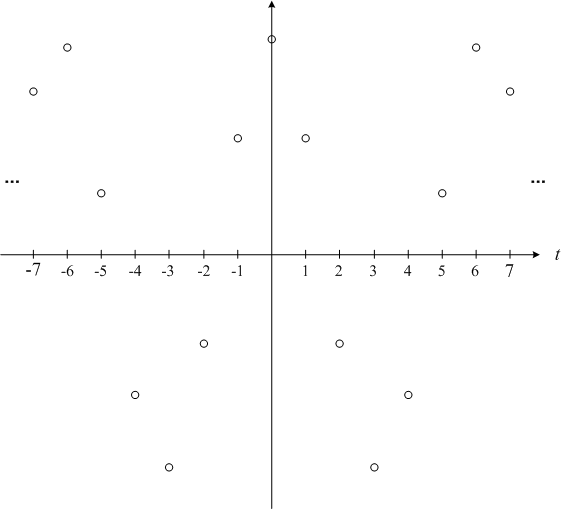As it happened, I started with a 1 rad/second cosine,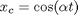,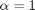:

t = linspace(-2.3*pi, 2.3*pi, 800);
alpha = 1;
x_c = cos(alpha * t);
plot(t, x_c, 'k')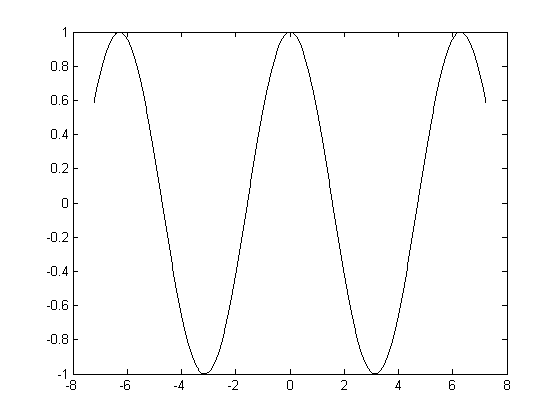And I sampled that signal to get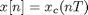with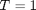so that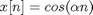.

T = 1;
n = -7:7;
nt = n * T;
x = cos(alpha*n*T);
hold on
plot(nt, x, 'ok')
hold off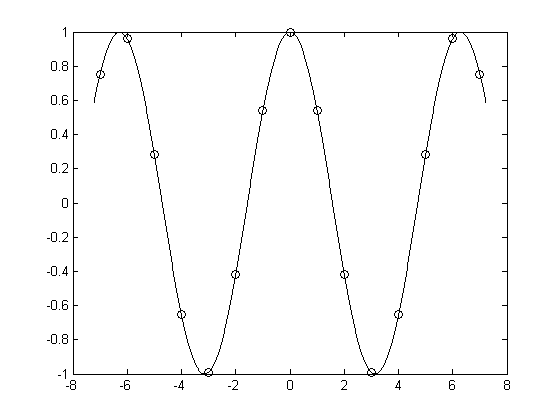But I could have started with a completely different frequency and still ended up with exactly the same samples. For example, let's see what happens when we try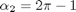:

alpha_2 = 2*pi - 1;
x2_c = cos(alpha_2 * t);
plot(t, x2_c, 'k')

x2 = cos(alpha_2 * n * T);
hold on
plot(nt, x2, 'ok')
hold off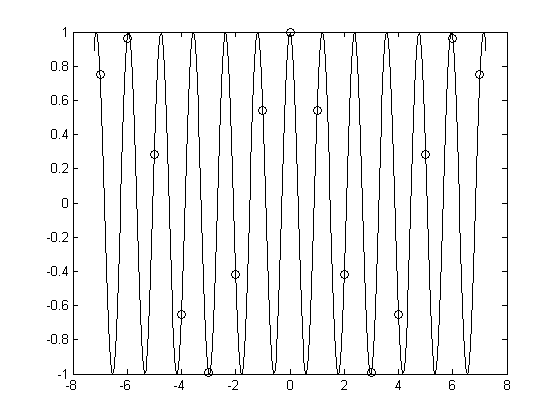The two sets of samples are the same (within floating-point round-off error):

max(abs(x - x2))
ans =

1.1657e-015



Let's look at this phenomenon in terms of the relationship between the continuous-time Fourier transform and the discrete-time Fourier transform (DTFT). Below is the continuous-time Fourier transform of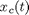.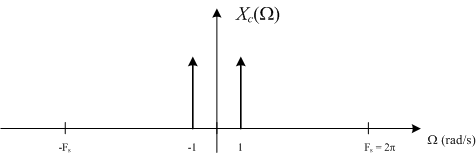The DTFT is a collection of copies of the continuous-time Fourier transform, spaced apart by the sampling frequency, and with the frequency axis scaled so that the sampling frequency becomes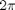. Here's the DTFT of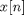.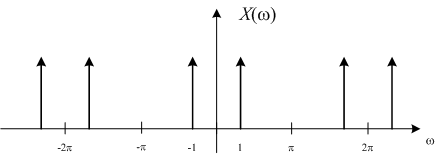Now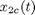has a higher frequency as you can see in the plot of its continuous-time Fourier transform: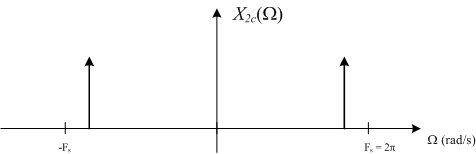But when you make a bunch of copies of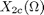spaced apart by the sampling frequency, you find that the DTFT of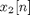is exactly the same as the DTFT of.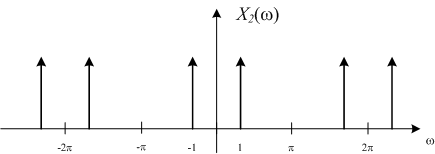Usually only a single period of the DTFT is plotted: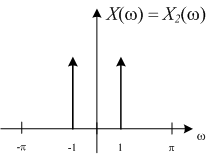In other words, when you use a sampling rate of, the frequencies 1 and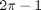are indistinguishable. This is called aliasing. In general, the continuous-time frequencyis indistinguishable from any other frequency of the form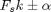, whereis an integer.

So far we've talked about the continuous-time Fourier transform, the discrete-time Fourier transform, their relationship, and a little bit about aliasing. Next time we'll bring the discrete Fourier transform (DFT) into the discussion. That's what the MATLAB function fft actually computes.

Published with MATLAB® 7.9

|

### 댓글

댓글을 남기려면 링크 를 클릭하여 MathWorks 계정에 로그인하거나 계정을 새로 만드십시오.